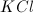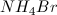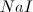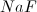# AP Chemistry : pH and POH of Strong Acids and Bases

## Example Questions

← Previous 1 3

### Example Question #1 : P H And Poh Of Strong Acids And Bases

Which of the following is a Lewis base?

BF3

Me3B

NH3

B2H6

NH3

Explanation:

NH3 should be the clear correct choice, since it is the only one having a pair of electrons that are available. The rest of the answer choices are all Lewis acids.

### Example Question #2 : P H And Poh Of Strong Acids And Bases

What is the defining characteristic of Bronsted-Lowry bases?

Dissociates in solution to give the OH– ion

Electron pair acceptor

Proton (H+) acceptor

Dissociates in solution to give the H+ ion

Proton (H+) donor

Proton (H+) acceptor

Explanation:

The definition of a Bronsted-Lowry base is a species that has the ability to gain, or accept a proton (H+). Dissociating in solution is part of the Arrhenius definition of acids and bases, and Lewis acid are electron pair acceptors.

### Example Question #161 : Reactions And Equilibrium

Which of the following can act as a Lewis base?

HF

BF3

CH3COOH

NH2NH2

NH2NH2

Explanation:

A Lewis base is an electron-pair donor. N atoms have a valence of 5, and in the NH2NH2 compound, it is only bonded to another N atom and 2 H, so it's only using 3 of its 5 valence electrons to form these bonds. Thus, each nitrogen has a pair of unbonded electrons and can act as a Lewis base.

### Example Question #162 : Reactions And Equilibrium

In the following equation, which is the conjugate base of HClO4?

HClO4 + H2O → ClO4– + H3O+

ClO4–

H3O+

H2O

none of the above

HClO4

ClO4–

Explanation:

The conjugate base of an acid will be the same compound, short one H atom. ClO4– is the only one that meets this criterion.

### Example Question #71 : Acid Base Reactions

Which of the following is a Lewis base?

CH2COOH

PH3

NH4+

CH3CH2CH2CH3

PH3

Explanation:

A Lewis base is an electron-pair donor. Only PH3 has a pair of nonbonding electrons and can act as a donor.

### Example Question #72 : Acid Base Reactions

Which of the following is the conjugate base of oxalic acid (H2C2O4)?

(C2O4) 2–

HC2O4–

HC2O4+

H2C2O4

HC2O4–

Explanation:

The conjugate base has one less H atoms and one unit greater negative charge because of this. Thus, the correct answer is HC2O4–

### Example Question #73 : Acid Base Reactions

Which of the following solutions will have a pH greater than 7?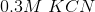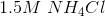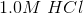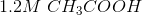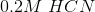Explanation:

HCN, HCl, CH3COOH, and NH4Cl are all acids (NH4+ is the ammonium ion). That only leaves KCN as the correct answer.

### Example Question #74 : Acid Base Reactions

BCl3 is a                     .

Arrhenius acid

Lewis acid

Bronsted-Lowry base

Lewis base

Bronsted-Lowry acid

Lewis acid

Explanation:

A Lewis acid is a species that can accept an electron pair. In the BCl3 molecule, B does not have a complete octet (3 covalent bonds, thus 6 electrons around it rather than 8). Thus, it can accept another electron pair, making it a Lewis acid.

### Example Question #75 : Acid Base Reactions

Which of the following compounds is a Lewis acid:,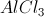,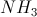,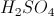?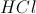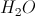Explanation:

A Lewis acid is a 2-electron acceptor, and a Lewis base is a 2-electron donor., while it is acidic, cannot accept two electrons, so it is not a Lewis acid.can accept two electrons, so it is a Lewis acid.is a Lewis base., like hydrochloric acid, is acidic, but cannot accept two electrons, so it is not a Lewis acid.

### Example Question #76 : Acid Base Reactions

Which of the following salts will result in an acidic solution?